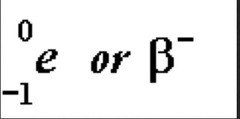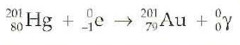# MCAT Physics: Atomic and Nuclear PhenomenaJosephine Mack
question

Photoelectric Effect

– When light of a sufficiently high frequency is incident on a metal in a vacuum, the metal ions emit electrons – liberated electrons produce a current
question

Threshold Frequency

– The minimum frequency of light that causes ejection of electrons (ft) – photoelectric effect is an all or nothing response
question

Photon

– light quanta in a light beam
question

Energy of each photon

E=hf – energy of each photon is proportional to light h= 6.626 x 10^-34 – energy of a photon increases with increasing frequency
question

Maximum kinetic energy of the ejected electron

where W is the work function of the metal in question – energy can be anywhere from 0 to Kmax
question

Work Function

– The minimum energy required to emit an electron – W=h ft (ft = threshold frequency) – activation energy in a sense
question

Absorption and Emission

– when an electron falls from a higher energy level to a lower energy level, a photon of light is emitted with an energy equal to the energy difference between the two orbitals
question

UV- Vis Spectroscopy

– looks at the absorption of light in the visible and ultraviolet range
question

Fluorescence

– if you excite a fluorescent substance with UV radiation it will begin to glow with visible light
question

Mass Defect

– mass of the nucleus is NOT the sum of the masses of all the protons and neutrons inside of it – The actual mass is slightly smaller due to matter being converted to energy E=mc^2 – because c is squared, a small amount of mass will yield a huge amount of energy
question

Strong Nuclear Force

– When protons and neutrons come together to form the nucleus they are attracted to each other by this force – binding energy allows the nucleons to bind together
question

Weak Nuclear Force

– much less strong than strong nuclear force
question

Isotopic notation

question

Fusion

– small nuclei combine to form a larger nucleus
question

Fission

– large nucleus splits into smaller nuclei – chain reaction – powers most commercial power plants
question

– naturally occurring spontaneous decay accompanied by the emission of specific particles Know these types of problems 1) the integer arithmetic of particle and isotope species 2) Radioactive half life problems 3) The use of exponential decay curves and decay constants
question

Isotope Decay Arithmetic and Nucleon Conversion

– the sum of the atomic numbers must be the same on both sides of the equation and the sum of the mass numbers must be the same on both sides as well
question

Alpha Decay

– Emission of an alpha particle – alpha particle consists of two protons, two neutrons and zero electrons – atomic number is 2 less and mass number is 4 less
question

Beta Decay

– emission of a beta particle which is an electron – emitted when a neutron decays into a proton, a beta particle and an antineutrino
question

Beta – Decay– neutron converted to a proton and a beta – particle – atomic number is one higher and mass number does not change
question

Beta + Decay

– a proton is converted into a neutron and a beta + particle – the atomic number will be one lower and the mass number will not change
question

Gamma Decay

– The emission of gamma rays which are high energy photons – carry now charge and simply lower the energy of the parent nucleus without changing the mass or atomic number
question

Electron Capture– capture an inner electron that combines with a proton to form a neutron – atomic number is one less but the mass number stays the same
question

Half Life

– the time it takes for half of the sample to decay
question

Exponential Decay

– the rate at which nuclei decay is proportional to the number that remain – gamma is decay constant
question

Exponential Decay